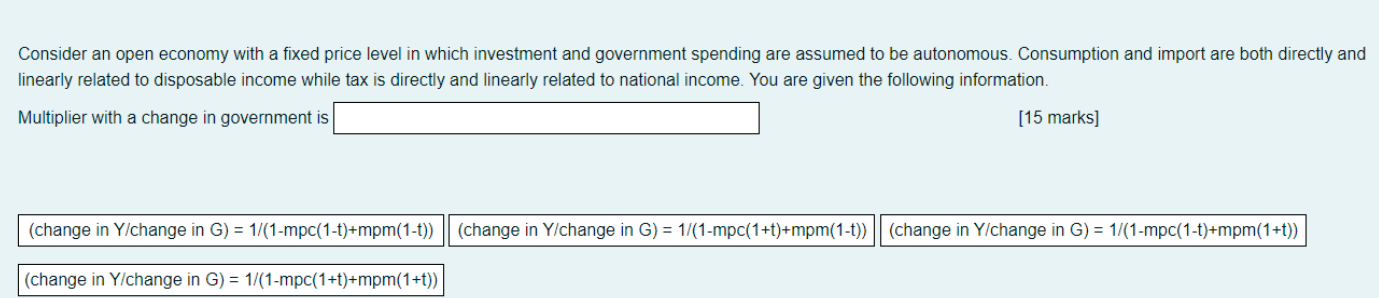### Create an Account

Home / Questions / Consider an open economy with a fixed price level in which investment and government spend...

# Consider an open economy with a fixed price level in which investment and government spending are assumed to be autonomous. Consumption and import are both directly and linearly related to disposable

Consider an open economy with a fixed price level in which investment and government spending are assumed to be autonomous. Consumption and import are both directly and linearly related to disposable income while tax is directly and linearly related to national income. You are given the following information. Multiplier with a change in government is [15 marks] (change in Y/change in G) = 1/(1-mpc(1-t)+mpm(1-t)) (change in Y/change in G) = 1/(1-mpc(1+t)+mpm(1-t)) || (change in Y/change in G) = 1/(1-mpc(1-t)+mpm(1+t)) (change in Y/change in G) = 1/(1-mpc(1+t)+mpm(1+t))May 08 2021 View more View LessSubscribe To Get Solution